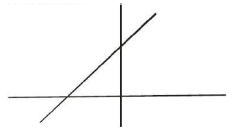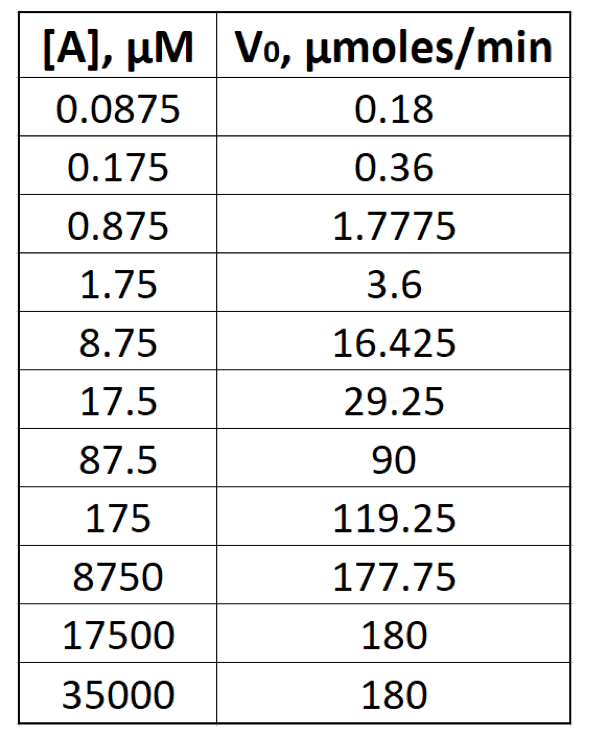Clutch Prep is now a part of Pearson
Ch. 6 - Enzymes and Enzyme KineticsWorksheetSee all chapters

# Lineweaver-Burk Plot

See all sections
Sections
Enzymes
Enzyme-Substrate Complex
Lock and Key Vs. Induced Fit Models
Optimal Enzyme Conditions
Activation Energy
Types of Enzymes
Cofactor
Catalysis
Electrostatic and Metal Ion Catalysis
Covalent Catalysis
Reaction Rate
Enzyme Kinetics
Rate Constants and Rate Law
Reaction Orders
Rate Constant Units
Initial Velocity
Vmax Enzyme
Km Enzyme
Michaelis-Menten Assumptions
Michaelis-Menten Equation
Lineweaver-Burk Plot
Michaelis-Menten vs. Lineweaver-Burk Plots
Shifting Lineweaver-Burk Plots
Calculating Vmax
Calculating Km
Kcat
Specificity Constant

Concept #1: Lineweaver-Burk Equation

Concept #2: Lineweaver-Burk Plot

Practice: A Lineweaver-Burk plot is used to:

Concept #3: X & Y-Intercepts of a Lineweaver-Burk Plot

Practice: To determine the Vmax from a Lineweaver-Burk plot you would:

Practice: A Lineweaver-Burk plot generates a line with the following formula: y = 0.3x + 0.4. What is the Km?

Practice: Consider the equation for the line on the following Lineweaver-Burk plot: y = 6x + 3A) What is the Km for the corresponding enzyme?

a) -2 mM

b) 18 mM

c) 2 mM

d) 9 mM

B) What is the Vmax for the corresponding enzyme?

a) 0.333 mM/s

b) 9 mM/s

c) -6 mM/s

d) 18 mM/s

Practice: Suppose the data on the right is plotted onto a Lineweaver-Burk plot to obtain a straight line.A) What is the value of the y-intercept of the line? ____________

B) What is the value of the x-intercept of the line? ____________